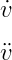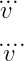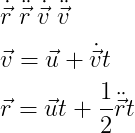# How to write dot derivative in LaTeX?

Mathematically, one or more dot symbols are used on a letter to denote a dot derivative. The default commands for this are \dot and \ddot and in which differential variables must be passed as arguments.

\documentclass{article}
\begin{document}
$\dot{v}$
$\ddot{v}$
\end{document}

Output :But, if you want to use more than two dots, you need to take the help of asmmath package. And in this case, I will use the \dddot and \ddddot commands.

\documentclass{article}
\usepackage{amsmath}
\begin{document}
$\dddot{v}$
$\ddddot{v}$
\end{document}

Output :Usually one or two dots are used. And while the use of three dots is very rare, the use of four dots is not at all.

## Vector dot derivatives in latex

This dot derivative is mostly used with vectors. For example

\documentclass{article}
\begin{document}
$\dot{\vec{r}}\; \ddot{\vec{r}}\; \dot{\vec{v}}\; \ddot{\vec{v}}$
$\vec{v}=\vec{u}+\dot{\vec{v}}t$
$\vec{r}=\vec{u}t+\frac{1}{2}\ddot{\vec{r}}t$
\end{document}

Output :I suggest you use the vv command of esvect package without using vec for the vector arrow. Because there are eight types of vector arrows present in this package. And each arrow will be positioned responsibly. And this is the best practice.

\documentclass{article}
\usepackage[b]{esvect}
\begin{document}
$\dot{\vv{v}}$
$\ddot{\vv{v}}$
$\vv{v} = \vv{u} + \dot{\vv{v}}t$
\end{document}

Output :#### Md Jidan Mondal

LaTeX expert with over 10 years of experience in document preparation and typesetting. Specializes in creating professional documents, reports, and presentations using LaTeX.